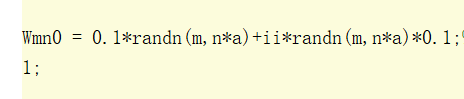# CVX objective function "maximize log2(1+rm)"

Recently, I’m learning to solve the convex optimization problem, which is shown in the picture.

I try to solve it by CVX. The code has no error it seems, but the results of CVX is not right. I’ve tried lots of methods but they didn’t work.
I don’t know what I did wrong, so I wonder if I need to transform my objective function “log2(1+rm)” into another form, since I’ve notice that when I run the code, there is a CVX Warning “Models involving “log” or other functions in the log, exp, and entropy
family are solved using an experimental successive approximation method.”

If you show us the solver and CVX output, we can say more. You may get that warning message whether or not the successive approximation method was used. But we can tell whether it actually was used by seeing the output.

As general advice, if you have Mosek available, use that as solver, with CVX 2.2. Otherwise, follow the advice at CVXQUAD: How to use CVXQUAD's Pade Approximant instead of CVX's unreliable Successive Approximation for GP mode, log, exp, entr, rel_entr, kl_div, log_det, det_rootn, exponential cone. CVXQUAD's Quantum (Matrix) Entropy & Matrix Log related functions

What evidence do you have that the CVX results are not right?

I tried the solver “SeDuMi” and CVX output just like figure.1.

When I chose Mosek as the solver, the result is shown in picture 2 and 3.
I found the two solvers got different results.

For the output, since it is different from the paper I’m simulating, so I think the results is not right.

The infeasibility certificate from Mosek is rather poor. Have you fiddled with some parameter settings? Changes to cvx_precision, or something like that?

Mosek warned about near zero elements. Perhaps the input data is poorly scaled,

I set Mosek as the specified solver and cvx_precision “best”. Maybe is there any wrong?

Maybe you mean the initial point I chose is not appropriate？

You haven’t shown us the input data. All we know about it is Mosek’s warning.

Sorry Mark, here is the input datas.

The data likes “Wmn0,rm0,t_k0,s_kn0,a_kn0” is my initial point, and the other inputs are constants.
Then “Wmn0” is generated randomly under constraints,Other inputs like “rm0,t_k0,s_kn0,a_kn0” are generated by satisfying the constraints from (35a) to (36b)

I think the initial point is feasible, but the result is error. Is there any wrong with me?

One obvious question is whether you were solving the same problem instance by SeDuMi (successive approximation method) and Mosek. Did you draw new random numbers when using Mosek vs. what was used with SeDuMi?

Anyhow, we still don’t know the numerical values of your input data.

NEVER set “cvx_precision best” with Mosek. Just leave it. It was discussed here many times. This setting produces parameter values not suitable for Mosek.

Oh yeap, every time I solved the problem by SeDuMi and Mosek, the inputs are different because it is generated randomly. But, if the input is a feasible point, whatever solver I used(maybe SeDuMi or Mosek) it will converge into a similar value. Isn’t it?

Oh sorry, I didn’t notice before. I will try it again. Thank you Michal

Try drawing a set of random numbers. Then run the exact same problem instance with both solvers. Then try a new set of random numbers, and run that exact same problem instance with both solvers.

For the output, since it is different from the paper I’m simulating, so I think the results is not right.

You shouldn’t expect to get the same answer (optimal objective value) unless all the input data is the same. Given that you are drawing random numbers, that would appear not to be the case.

OK, I’ll try the way. Thank you so so so much Mark.
Emmm, by the way, I still have a question that, sometimes when CVX is running, the Status is failed and the Optimal value(cvx_optval) is NAN, but the output in my code also has a determined value. I don’t quite understand it.

Emm, Michal, one more question. I wonder that if I can specify the solving method in CVX, like solve the problem using a solver via interior-point method.

There isn’t any other way in Mosek for your problem.

If CVX status is failed, all variable values are meaningless.

Emmm, I mean can I specify Mosek solver uses interior_point method to solve my problem? Or Mosek will find a most appropriate method by itself to calculate it.

OK, I got it. Thank you Mark!!!
One more quetion, can I specify Mosek solver uses interior_point method to solve my problem(like: by using some specific statement)? Or Mosek will find a most appropriate method by itself to calculate it.